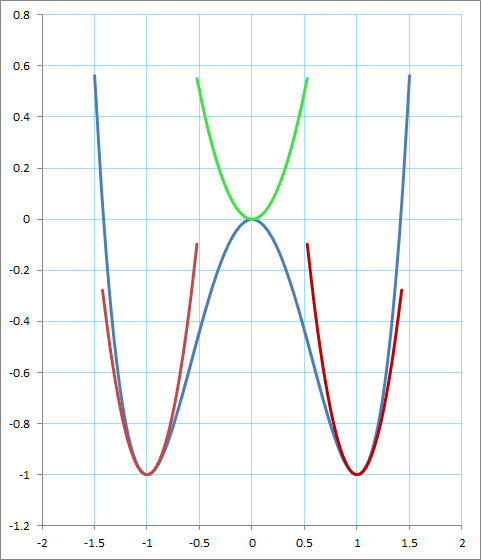# Frequency of oscillations

## Homework Statement

A particle moves in 1D in a potential of the form $$U=Ax^2+Bx^4$$ where A can be either positive or negative. Find the equilibrium points and the frequency of small oscillations.

## The Attempt at a Solution

So the equilibrium points are obtained by setting the derivative of U equal to 0. If A is positive the equilibrium point is 0 and the frequency is ##\sqrt{2A/m}##. If A is negative the equilibrium points (stable equilibrium) are ##\pm\sqrt{-A/2B}##. Now in the book, they say that the frequency of the oscillations here is equal to ##\sqrt{-A/m}## but I don't get this answer. This is what I did: $$\ddot{x}=-2Ax-4Bx^3$$ I will call the equilibrium point of the case when A is negative, simply ##x## and the small deviation ##a## so the equation becomes: $$\ddot{x}+\ddot{a}=-2A(x+a)-4B(x+a)^3$$ $$\ddot{x}+\ddot{a}=-2Ax-2Aa-4Bx^3(1+a/x)^3$$ $$\ddot{x}+\ddot{a}=-2Ax-2Aa-4Bx^3(1+3a/x)$$ and using the fact that x is the equilibrium point, we get: $$\ddot{a}=-2Aa-12aBx^2$$ and we know that here ##x=\pm\sqrt{-A/2B}## so we get $$\ddot{a}=-2Aa-12aB(-A/2B)$$ $$\ddot{a}=4Aa$$ so the osciallation frequency would be ##\sqrt{-4A/m}## (I ignored the mass in the equations). What am I doing wrong, why do I get that 4 there? Thank you!

mjc123
Homework Helper
I agree with your answers; perhaps there is a mistake in the book.
(Strictly, what you have calculated is the angular frequency ω (rad/s). The frequency of oscillation (cycles/s) is ω/2π.)

BvU
Homework Helper
Hi,

So you replace ##U(x)## by ##U(a) = 2A a^2##

Who says you do anything wrong ? The factor 2 appearing in ##U(a)## makes it logical you get a factor ##\sqrt 2## in the frequency.

SO I suspect an error in the book answer...

The red parabolas (this is for (##A=-2,\ \ B= 1##) at ##\pm 1## are sharper than the green (for ##A=+2,\ \ B= 0##) at 0